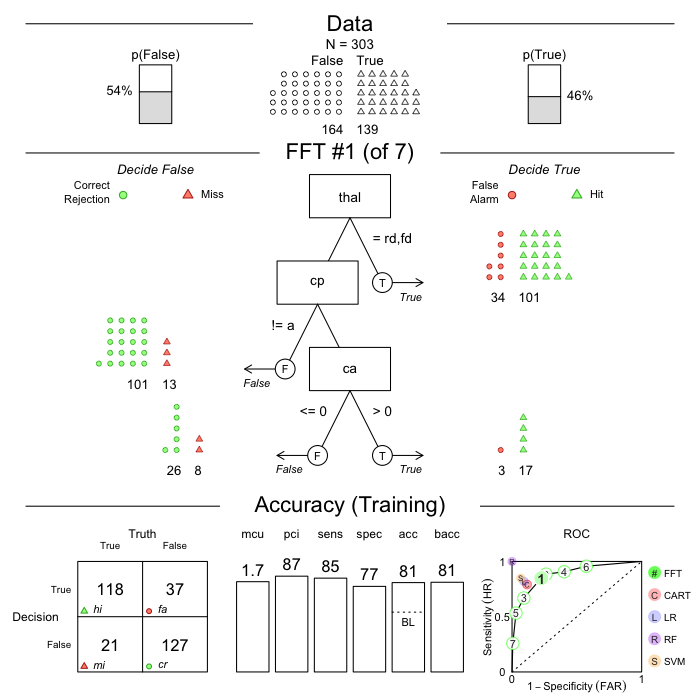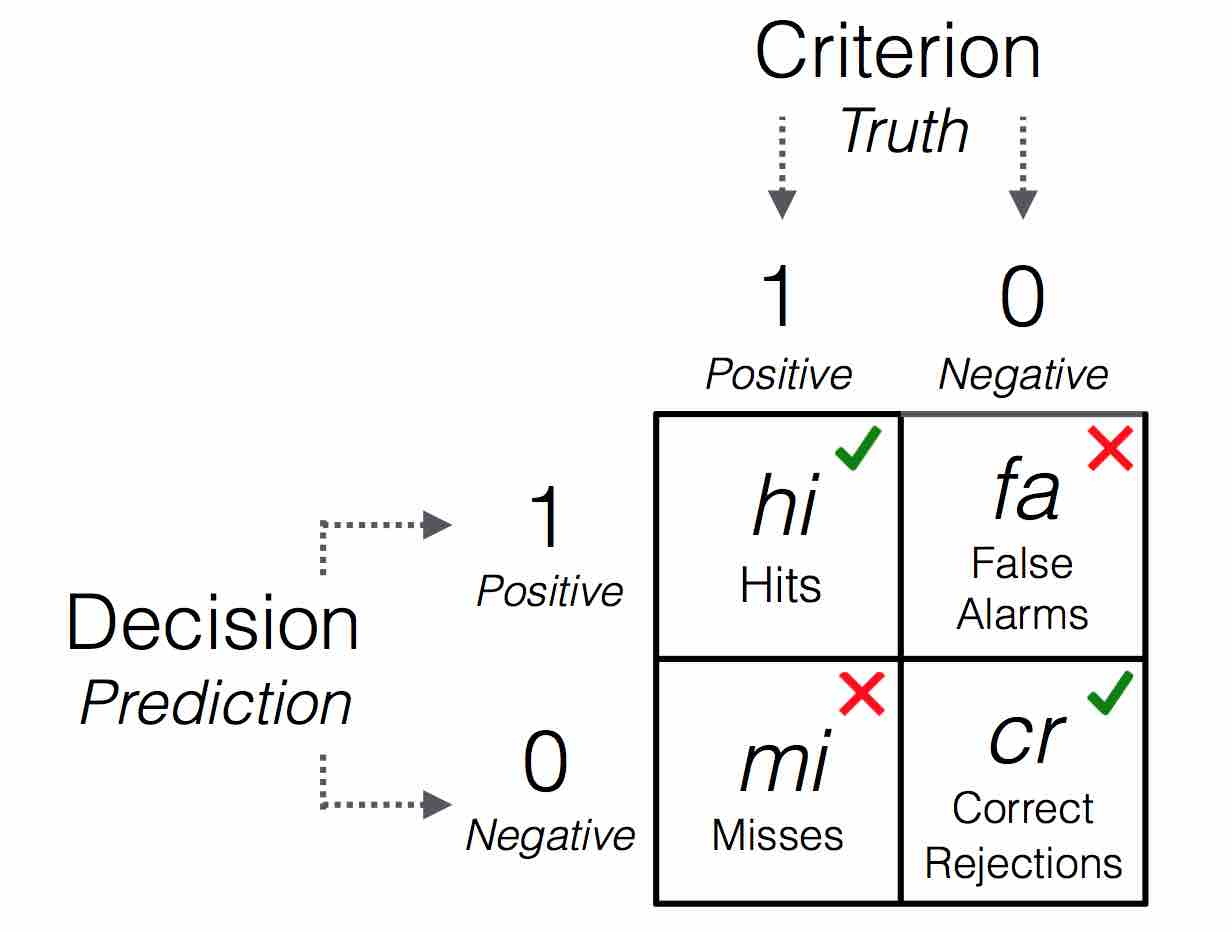# Accuracy statistics in FFTrees

## Accuracy Statistics in FFTrees

In this vignette, we cover how accuracy statistics are calculated for FFTs and the FFTrees package (as described in Phillips, Neth, Woike, & Gaissmaier, 2017). Most of these measures are not specific to FFTs and can be used for any classification algorithm.

First, let’s examine the accuracy statistics from an FFT predicting heart disease:

# Create an FFTrees object predicting heart disease:
heart.fft <- FFTrees(formula = diagnosis ~.,
data = heartdisease)

Running this FFTrees() function call yields a new FFTrees object heart.fft. Both printing or plotting this object for a particular dataset and tree yields corresponding accuracy and frugality statistics. For now, we simply plot the best training tree:

plot(heart.fft, tree = "best.train")Figure 1: Example FFT for the heartdisease data.

We notice a 2x2 table in the bottom-left corner of the plot: This is a 2 x 2 matrix or confusion table (see Wikipedia or Neth et al., 2021 for details). A wide range of accuracy measures can be derived from this seemingly simple matrix. Here is a generic version of a confusion table:Figure 2: A 2x2 matrix illustrating the frequency counts of 4 possible outcomes.

A 2 x 2 matrix cross-tabulates the decisions of the algorithm (rows) with actual criterion values (columns) and contains counts of observations for all four resulting cells. Counts in cells a and d refer to correct decisions due to a match between predicted and criterion values, whereas counts in cells b and c refer to errors due to the mismatch between predicted and criterion values. Both correct decisions and errors come in two types:

• Correct decisions in cell hi represent hits, positive criterion values correctly predicted to be positive, and cell cr represents correct rejections, negative criterion values correctly predicted to be negative.

• As for errors, cell fa represents false alarms, negative criterion values erroneously predicted to be positive, and cell mi represents misses, positive criterion values erroneously predicted to be negative.

Given this structure, an accurate decision algorithm aims to maximize the frequencies in cells hi and cr while minimizing those in cells fa and mi.

Output Description Formula
hi Number of hits $$N(\text{Decision} = 1 \land \text{Truth} = 1)$$
mi Number of misses $$N(\text{Decision} = 0 \land \text{Truth} = 1)$$
fa Number of false-alarms $$N(\text{Decision} = 1 \land \text{Truth} = 0)$$
cr Number of correct rejections $$N(\text{Decision} = 0 \land \text{Truth} = 0)$$
N Total number of cases $$\text{N} = \text{hi} + \text{mi} + \text{fa} + \text{cr}$$

Table 1: Definitions of the frequency counts in a 2x2 confusion table. The notation $$N()$$ means number of cases (or frequency counts).

### Conditional accuracy statistics

The first set of accuracy statistics are based on subsets of the data. These subsets result from focusing on particular cases of interest and computing conditional probabilities based on them. Given the 2x2 structure of the confusion table, measures can be conditional on either algorithm decisions (positive predictive vs. negative predictive values) or criterion values (sensitivity vs. specificity). In other words, these measures are conditional probabilities that are based on either the rows or columns of the confusion table:

Output Description Formula
sens Sensitivity $$p(\text{Decision} = 1 \ \vert\ \text{Truth} = 1) = \text{hi} / (\text{hi} + \text{mi})$$
spec Specificity $$p(\text{Decision} = 0 \ \vert\ \text{Truth} = 0) = \text{cr} / (\text{cr} + \text{fa})$$
far False alarm rate $$1 - \text{Specificity}$$ (spec)
ppv Positive predictive value $$p(\text{Truth} = 1 \ \vert\ \text{Decision} = 1) = \text{hi} / (\text{hi} + \text{fa})$$
npv Negative predictive value $$p(\text{Truth} = 0 \ \vert\ \text{Decision} = 0) = \text{cr} / (\text{cr} + \text{mi})$$

Table 2: Conditional accuracy statistics based on either the rows or columns of a 2x2 confusion table.

The sensitivity (aka. hit-rate) is defined as $$sens = hi/(hi + mi)$$ and represents the percentage of cases with positive criterion values that were correctly predicted by the algorithm. Similarly, specificity (aka. correct rejection rate, or the complement of the false alarm rate) is defined as $$spec = cr/(fa + cr)$$ and represents the percentage of cases with negative criterion values correctly predicted by the algorithm.

The positive-predictive value $$ppv$$ and negative predictive value $$npv$$ are the flip-sides of $$sens$$ and $$spec$$, as they are conditional accuracies based on decision outcomes (rather than on true criterion values).

### Aggregate accuracy statistics

Additional accuracy statistics are based on all four cells in the confusion table:

Output Description Formula
acc Accuracy $$(\text{hi} + \text{cr}) / (\text{hi} + \text{mi} + \text{fa} + \text{cr})$$
bacc Balanced accuracy $$\text{sens} \times .5 + \text{spec} \times .5$$
wacc Weighted accuracy $$\text{sens} \times w + \text{spec} \times (1 - w)$$
bpv Balanced predictive value $$\text{ppv} \times .5 + \text{npv} \times .5$$
dprime D-prime $$z_{\text{sens}} - z_{\text{far}}$$

Table 3: Aggregate accuracy statistics based on all four cells of a 2x2 confusion table.

Overall accuracy (acc) is defined as the overall percentage of correct decisions ignoring the difference between hits and correct rejections. The more specific measures $$bacc$$ and $$wacc$$ are averages of sensitivity and specificity, while $$bpv$$ is an average of predictive values. The $$dprime$$ measure is the difference in standardized ($$z$$-score) transformed $$sens$$ and $$far$$ (see Luan, Schooler, & Gigerenzer, 2011 for the relation between FFTs and signal detection theory, SDT).

### Speed and frugality statistics

The next two statistics measure the speed and frugality of a fast-and-frugal tree (FFT). Unlike the accuracy statistics above, they are not based on the confusion table. Rather, they depend on how much information FFTs use to make their predictions or decisions.

Output Description Formula
mcu Mean cues used: Average number of cue values used in making classifications, averaged across all cases
pci Percentage of cues ignored: Percentage of cues ignored when classifying cases $$N(\text{cues in data}) - \text{mcu}$$

Table 4: Measures to quantify the speed and frugality of FFTs.

To see exactly where these statistics come from, let’s look at the results for heart.fft (Tree #1):

heart.fft
#> FFTrees
#> - Trees: 7 fast-and-frugal trees predicting diagnosis
#> - Outcome costs: [hi = 0, mi = 1, fa = 1, cr = 0]
#>
#> FFT #1: Definition
#>  If thal = {rd,fd}, decide True.
#>  If cp != {a}, decide False.
#>  If ca > 0, decide True, otherwise, decide False.
#>
#> FFT #1: Training Accuracy
#> Training data: N = 303, Pos (+) = 139 (46%)
#>
#> |          | True + | True - | Totals:
#> |----------|--------|--------|
#> | Decide + | hi 118 | fa  37 |     155
#> | Decide - | mi  21 | cr 127 |     148
#> |----------|--------|--------|
#>   Totals:       139      164   N = 303
#>
#> acc  = 80.9%   ppv  = 76.1%   npv  = 85.8%
#> bacc = 81.2%   sens = 84.9%   spec = 77.4%
#>
#> FFT #1: Training Speed, Frugality, and Cost
#> mcu = 1.73,  pci = 0.87,  E(cost) = 0.191

According to this output, Tree #1 has mcu = 1.73 and pci = 0.87. We can easily calculate these measures directly from the x$levelout output of an FFTrees object. This object contains the tree level (i.e., node) at which each case was classified: # A vector of levels/nodes at which each case was classified: heart.fft$trees$decisions$train$tree_1$levelout
#>    1 3 1 2 2 2 3 3 1 1 1 2 1 1 1 2 1 3 2 2 2 2 2 1 1 2 2 2 3 1 2 1 2 1 2 3 1
#>   1 1 2 1 1 2 2 3 1 2 1 2 2 2 1 3 2 1 1 1 1 2 2 1 2 1 2 1 1 2 1 1 2 2 1 1 1
#>   3 2 1 2 2 1 3 3 2 1 2 2 2 2 3 2 3 1 1 2 2 1 1 1 2 3 3 2 3 2 1 1 1 1 1 1 1
#>  3 1 1 1 1 2 3 1 1 1 1 2 1 2 2 1 1 2 3 1 1 2 3 2 2 1 1 1 2 2 1 2 1 1 2 1 2
#>  2 2 1 3 1 1 3 3 1 1 1 1 1 3 2 3 2 1 2 2 1 2 1 1 3 3 1 1 1 1 2 2 1 1 2 1 3
#>  2 1 1 1 1 2 1 1 3 2 3 2 3 2 2 3 3 1 1 1 1 1 1 2 3 2 1 2 1 3 1 2 3 3 3 2 2
#>  2 1 3 2 3 2 3 3 2 3 2 2 2 3 1 1 2 2 2 2 3 2 2 3 1 3 1 2 1 1 1 2 3 2 3 2 2
#>  1 2 2 2 2 3 1 3 1 1 2 1 1 1 3 2 1 2 2 2 3 1 2 1 2 1 1 1 1 1 2 1 2 1 1 3 2
#>  1 1 1 1 1 2 2

Now, to calculate mcu (the mean number of cues used), we simply take the mean of this vector:

# Calculate the mean number or cues used (mcu):
mean(heart.fft$trees$decisions$train$tree_1$levelout) #>  1.732673 Now that we know where mcu comes from, computing pci (i.e., the percentage of cues ignored) is just as simple — it’s just the total number of cues in the dataset minus mcu, divided by the total number of cues in the data: # Calculate pci (percentage of cues ignored) as # (n.cues - mcu) / n.cues): n.cues <- ncol(heartdisease) (n.cues - heart.fft$trees$stats$train\$mcu) / n.cues
#>  0.8762376

There is a wide range of additional measures that can be used to quantify classification performance. Most of these can easily be computed from the numerical information provided in an FFTrees object. For alternative measures based on the frequency counts of a 2x2 matrix, see Table 3 of Neth, Gradwohl, Streeb, Keim, & Gaissmaier (2021).

## Vignettes

Here is a complete list of the vignettes available in the FFTrees package:

Vignette Description
Main guide: FFTrees overview An overview of the FFTrees package
1 Tutorial: FFTs for heart disease An example of using FFTrees() to model heart disease diagnosis
2 Accuracy statistics Definitions of accuracy statistics used throughout the package
3 Creating FFTs with FFTrees() Details on the main function FFTrees()
4 Specifying FFTs directly How to directly create FFTs with my.tree without using the built-in algorithms
5 Visualizing FFTs with plot() Plotting FFTrees objects, from full trees to icon arrays
6 Examples of FFTs Examples of FFTs from different datasets contained in the package